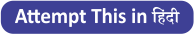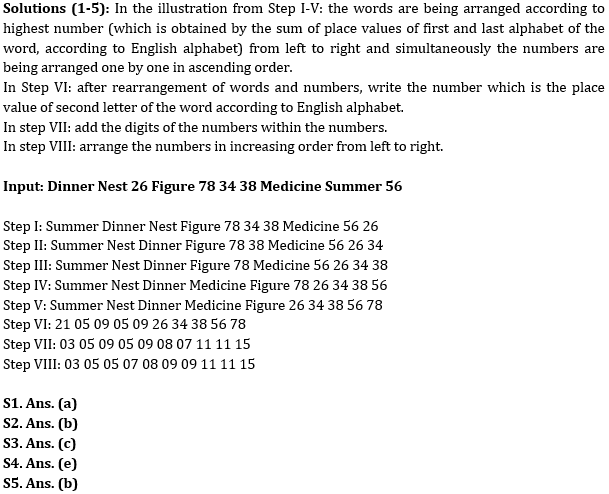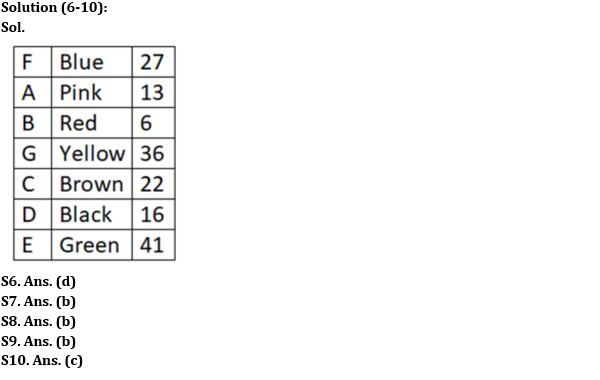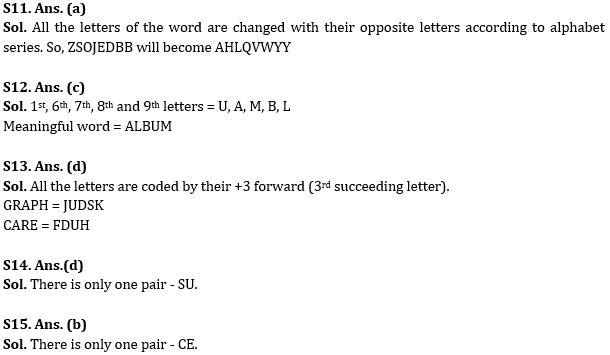Latest Banking jobs   »

# Reasoning Ability Quiz For RBI Assistant/ ESIC UDC Mains 2022- 18th April

Directions (1-5): Directions: A word and number arrangement machine when given an input line of words and numbers rearranges them following a particular rule in each step. The following is an illustration of an input rearrangement.Input: Current True 28 30 Given 24 Situation 48 Triangles 52
Step I: Triangles Current True 28 30 Given Situation 48 52 24
Step II: Triangles Situation Current True 30 Given 48 52 24 28
Step III: Triangles Situation True Current Given 48 52 24 28 30
Step IV: Triangles Situation True Current Given 52 24 28 30 48
Step V: Triangles Situation True Current Given 24 28 30 48 52
Step VI: 18 09 18 21 09 24 28 30 48 52
Step VII: 09 09 09 03 09 06 10 03 12 07
Step VIII: 03 03 06 07 09 09 09 09 10 12
Step VIII is the output of the above input

Input: Dinner Nest 26 Figure 78 34 38 Medicine Summer 56

Q1. In the Step V, which element will be sixth to the right of seventh element from the right end?
(a) 78
(b) Nest
(c) 56
(d) 38
(e) None of these

Q2. What would be the sum of all the numbers in the penultimate step?
(a) 95
(b) 83
(c) 75
(d) 88
(e) None of these

Q3. Which element is second to the right of third element from left end in step IV?
(a) 78
(b) Nest
(c) Figure
(d) Medicine
(e) None of these

Q4. What is the sum of first four numbers from left end in last step?
(a) 12
(b) 18
(c) 14
(d) 10
(e) 20

Q5. Which element is third to the left of third element from the right end in step III?
(a) 26
(b) 78
(c) Medicine
(d) Dinner
(e) None of these

Directions (6-10): Study the following data carefully and answer the questions accordingly.
Seven boxes A, B, C, D, E, F, and G of seven different colors i.e., Blue, Black, Brown, Red, Pink, Yellow, and Green are placed one above the other but not necessarily in the same order. Each box contains a different number of pencils i.e., 6, 13, 16, 22, 27, 36, and 41 but not necessarily in the same order.
F contains less number of pencils than E. B which is not green, contains less number of pencils than A. The pink box is placed above the green box. The black box contains a square number of pencils and placed near to C which contains an even number of pencils. Three boxes are placed between F which is blue in color and the brown box. E is not yellow. A is placed four places above D which is not green. Green box is placed near the box that contains 16 pencils. Two boxes are placed between the blue box and the yellow box which contains 36 pencils. Only two boxes are placed between C which is brown and the pink box that contains 13 pencils.

Q6. Which of the following boxes contains 27 pencils?
(a) D
(b) Green box
(c) G
(d) Blue box
(e) None of these

Q7. How many boxes are placed below the red box?
(a) One
(b) Four
(c) Two
(d) Three
(e) None of these

Q8. Which of the following boxes is green?
(a) G
(b) E
(c) D
(d) B
(e) None of these

Q9. Which of the following statement is correct?
(a) B contains more pencils than C
(b) Pink box does not contain the least number of pencils
(c) Two boxes are placed below the black box
(d) The box containing 36 pencils is placed immediately above the red box
(e) None is correct

Q10. Four among the following five are same in a certain way and related to a group. Which among the following does not belong to the group?
(a) D, 36
(b) E, brown
(c) B, 36
(d) C, 6
(e) G, pink

Q11. In a certain code, XUTPOJDB is written as CFGKLQWY and VUMFCBB is written as EFNUXYY. How would ZSOJEDBB be written in that code language?
(a) AHLQVWYY
(b) YYAHVWLQ
(c) HAQVLWYY
(d) AHLQUWYY
(e) None of these

Q12. How many meaningful English words can be formed from the first, sixth, seventh, eighth and ninth letters of the word UNSCRAMBLE using them in different sequence only once?
(a) Three
(b) Two
(c) One
(d) Six
(e) Four

Q13. In a certain code, ‘GRAPH’ is written as ‘JUDSK’. How is ‘CARE’ written in that code?
(a) FZTO
(b) FZUU
(c) FZZD
(d) FDUH
(e) FZDU

Q14. How many such pairs of letters are there in the word THESAURUS each of which has as many letters between them in the word as in the English alphabet (both forward and backward direction)?
(a) Four
(b) Two
(c) None
(d) One
(e) None of these

Q15. How many such pairs of letters are there in the word IRREVERENCE each of which has as many letters between them (in both forward and backward direction) in the word as in the English alphabet?
(a) Four
(b) One
(c) Five
(d) Two
(e) None of these

Solutions#### Congratulations!Download Hindu Review of October 2021: Free PDF